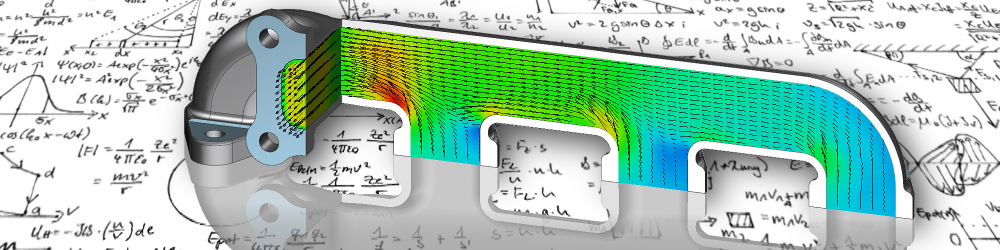•• 1

# (In)compressible flow

Formally speaking, all fluids are compressible, which means that the density depends on the existing pressure. This relationship of thermodynamic quantities is described by the equations of state.

## incompressible flows

In practice, the pressure dependence of the density, even with flowing gases, is only of importance if the flow velocity approaches the speed of sound. At slow flows, the limit is a Mach number of 0.3, the differences in density in the flow field are small. To simplify matters, the fluid can be regarded as incompressible. The density is then no longer a searched quantity, but becomes a given substance property. This also decouples the energy equation, which means that temperature influences can either be ignored or considered separately. From the point of view of numerical effort, this is advantageous if only the flow is to be considered individually.

Differences in density due to temperature, which, as with natural convection, cause or at least influence the flow, cannot be described in this way. This is achieved using the Boussinesq approximation. With respect to a reference temperature, the dependence of the density on the temperature is established via a coefficient of thermal expansion. Thus the impulse equation is again coupled to the energy equation.

## compressible flows

At flow velocities from a Mach number of 0.3, however, the density changes in the fluid must be taken into account. It then follows from the equations of state that temperature and pressure changes are also significant and must therefore be taken into account. A simplification of the basic equations is therefore no longer possible,

• the continuity equation
• the impulse equation
• the energy equation and
• the equation of state

must be solved simultaneously. Compared to the simplified assumption of incompressible flow, this means double the effort. This system of equations could only be reduced in its complexity by further assumptions, such as an isotropic flow.Warmesberg 24, 9450 Altstätten, Switzerland

+41 79 471 1612